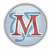Maxima
Operating system: Windows
Category: Education
Review rating:
Official page: Maxima
Wikipedia: Maxima

#### Description

Maxima – a computer algebra system to work with symbolic and numerical expressions. The software contains a set of tools for differentiation, integration, expansion in series, Laplace transform, solution of ordinary differential equations, systems of linear equations etc. Maxima enables a high-precision numerical calculations using whole numbers, exact fraction or variable-precision floating-point numbers. The software also allows you to build 2 and 3 dimensional graphics functions or statistical data.

#### Main features:

• Work with symbolic and numerical expressions
• Support of various types of of calculations
• The ability to build 2 and 3 dimensional graphicsVersion:
5.44
Architecture:
Language:
English